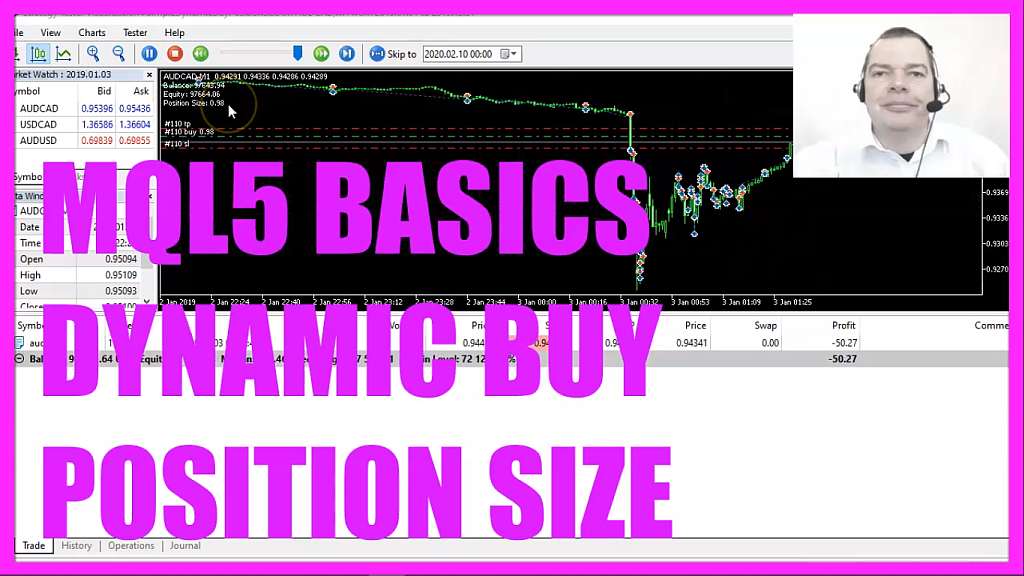In this video, we are going to find out how to dynamically calculate position sizes for buy trades, in this case, based on the equity, so let’s find out how to do that with mql5.
To get started please click on the little button here or press F4 on your keyboard, now you should see the Metaeditor window and here you want to click on file, new file, expert advisor from template, continue, I will call this file simple dynamic buy position size, click on continue, continue and finish, now you can delete everything above the on tick function and the two comment lines here.
We start with an include statement to include the file trade dot mqh, this file comes with mql5 and we are going to use it to open positions.
To do that we need to create an instance of the class ctrade, we will call that one trade and inside of the on tick function we need to calculate a few things, first we want to calculate the ask price, that is a double value because it’s a floating type, the variable name is ask and we can calculate the ask price by using symbol info double, for the current symbol on the chart, we use symbol underscore ask and with normalize double and underscore digits we automatically calculate the right number of digits behind the dot for the currency pair on the chart.
Afterwards, we want to calculate the equity, the equity is what is left over after we have paid for costs and open trades and to calculate it we use account info double, the parameter we use is account underscore equity – all in capital letters.
Let’s repeat that for the balance. The account balance is calculated by using account info double and here is the parameter, account underscore balance, this one is also in capital letters.
Afterwards, we are going to calculate the position size, this is a double value and we use normalize double to cut the results to two digits behind the dot because when we divide the equity by 100,000 the result might have six to eight digits behind the dot and that would cause an error because when we try to open a new position we would get a journal entry that the position size is wrong and this is going to prevent that.
So let’s check the conditions!
First, we want to know if the equity is equal or bigger than the balance, the second condition would be, we want to know if the return value for positions total is zero, if that is the case we don’t have any open positions and that is when we use trade dot buy to open a buy trade for the dynamically calculated position size that we have calculated here.
Finally, we use the comment statement to create an output on the chart that will show us the balance, the equity and the calculated position size.